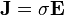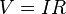# Electrical conductor

In science and engineering, an electrical conductor is a material that contains movable electric charges. In metallic conductors, such as copper or aluminum, the movable charged particles are electrons. Positive charges may also be mobile in the form of atoms in a lattice missing electrons (called "holes") or ions, such as in the electrolyte of a battery.

## Details

The following applies to cases involving direct current only. When the direction of voltage/current alternates, other effects (inductance and capacitance) come into play also.

All conductors contain electric charges which will move when an electric potential difference (measured in volts) is applied across separate points on the material. This flow of charge (measured in amperes) is what is meant by electric current. In most materials, the rate of current is proportional to the voltage (Ohm's law,) provided the temperature remains constant and the material remains in the same shape and state. The ratio between the voltage and the current is called the resistance (measured in ohms) of the object between the points where the voltage was applied. The resistance across a standard mass (and shape) of a material at a given temperature is called the resistivity of the material. The inverse of resistance and resistivity is conductance and conductivity. Some good examples of conductors are metal.

Most familiar conductors are metallic. Copper is the most common material for electrical wiring (silver is the best but expensive), and gold for high-quality surface-to-surface contacts. However, there are also many non-metallic conductors, including graphite, solutions of salts, and all plasmas.

Non-conducting materials lack mobile charges, and so resist the flow of electric current, generating heat. In fact, all materials offer some resistance and warm up when a current flows. Thus, proper design of an electrical conductor takes into account the temperature that the conductor needs to be able to endure without damage, as well as the quantity of electrical current. The motion of charges also creates an electromagnetic field around the conductor that exerts a mechanical radial squeezing force on the conductor. A conductor of a given material and volume (length x cross-sectional area) has no real limit to the current it can carry without being destroyed as long as the heat generated by the resistive loss is removed and the conductor can withstand the radial forces. This effect is especially critical in printed circuits, where conductors are relatively small and close together, and inside an enclosure: the heat produced, if not properly removed, can cause fusing (melting) of the tracks.

Since all conductors have some resistance, and all insulators will carry some current, there is no theoretical dividing line between conductors and insulators. However, there is a large gap between the conductance of materials that will carry a useful current at working voltages and those that will carry a negligible current for the purpose in hand, so the categories of insulator and conductor do have practical utility.

Thermal and electrical conductivity often go together (for instance, most metals are both electrical and thermal conductors). However, some materials are practical electrical conductors but are not good thermal conductors.

## Electrical conductivity

Electrical conductivity or specific conductivity is a measure of a material's ability to conduct an electric current. When an electrical potential difference is placed across a conductor, its movable charges flow, giving rise to an electric current. The conductivity σ is defined as the ratio of the current density$\mathbf{J}$ to the electric field strength$\mathbf{E}$:$\mathbf{J} = \sigma \mathbf{E}$

It is also possible to have materials in which the conductivity is anisotropic, in which case σ is a 3×3 matrix (or more technically a rank-2 tensor) which is generally symmetric.

Conductivity is the reciprocal (inverse) of electrical resistivity and has the SI units of siemens per meter (S·m-1), such as, if the electrical conductance between opposite faces of a 1-meter cube of material is 1 Siemens then the material's electrical conductivity is 1 Siemens per meter. Electrical conductivity is commonly represented by the Greek letter σ, but κ or γ are also occasionally used.

An EC meter is normally used to measure conductivity in a solution.

### Classification of materials by conductivity

Materials can be classified based on their electrical conductivity, as follows.

• A conductor such as a metal has high conductivity.
• An insulator like glass or a vacuum has low conductivity.
• The conductivity of a semiconductor is generally intermediate, but varies widely under different conditions, such as exposure of the material to electric fields or specific frequencies of light, and, most important, with temperature and composition of the semiconductor material.

The degree of doping in solid state semiconductors makes a large difference in conductivity. More doping leads to higher conductivity. The conductivity of a solution of water is highly dependent on its concentration of dissolved salts and sometimes other chemical species which tend to ionize in the solution. Electrical conductivity of water samples is used as an indicator of how salt-free or impurity-free the sample is; the purer the water, the lower the conductivity.

## Power engineering

In power engineering, a conductor is a piece of metal used to conduct electricity, known colloquially as an electrical wire.

### Conductor size

In many countries, conductors are measured by their cross section in square millimeters.

However, in the United States, conductors are measured by American wire gauge for smaller ones, and circular mils for larger ones. In some poor countries they have overloaded wires going into one circuit.

### Conductor materials

Of the metals commonly used for conductors, copper, has a high conductivity. Silver is more conductive, but due to cost it is not practical in most cases. However, it is used in specialized equipment, such as satellites, and as a thin plating to mitigate skin effect losses at high frequencies. Because of its ease of connection by soldering or clamping, copper is still the most common choice for most light-gauge wires. Aluminum has been used as a conductor in housing applications for cost reasons. It is actually more conductive than copper when compared by unit weight, but it has technical problems related to heat and compatibility of metals.

## Conductor voltage

The voltage on a conductor is determined by the connected circuitry and has nothing to do with the conductor itself. Conductors are usually surrounded by and/or supported by insulators and the insulation determines the maximum voltage that can be applied to any given conductor.

Voltage of a conductor "V" is given by$V ={I}{R}$

where

I is the current, measured in amperes
V is the potential difference measured in volts
R is the resistance measured in ohms

## Conductor ampacity

The ampacity of a conductor, that is, the amount of current it can carry, is related to its electrical resistance: a lower-resistance conductor can carry more current. The resistance, in turn, is determined by the material the conductor is made from (as described above) and the conductor's size. For a given material, conductors with a larger cross-sectional area have less resistance than conductors with a smaller cross-sectional area.

For bare conductors, the ultimate limit is the point at which power lost to resistance causes the conductor to melt. Aside from fuses, most conductors in the real world are operated far below this limit, however. For example, household wiring is usually insulated with PVC insulation that is only rated to operate to about 60°C, therefore, the current flowing in such wires must be limited so that it never heats the copper conductor above 60°C, causing a risk of fire. Other, more expensive insulations such as Teflon or fiberglass may allow operation at much higher temperatures.

The American wire gauge article contains a table showing allowable ampacities for a variety of copper wire sizes.

## Isotropy

If an electric field is applied to a material, and the resulting induced electric current is in the same direction, the material is said to be an isotropic electrical conductor. If the resulting electric current is in a different direction from the applied electric field, the material is said to be an anisotropic electrical conductor!.